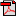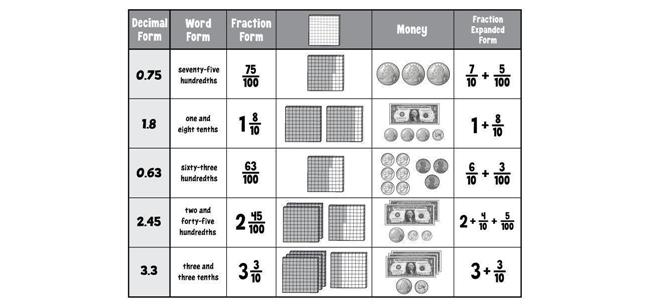# Lesson 4: Decimals to Thousandths

## Getting Started

Materials: glue or glue stick, Interactive Notebook, scissors
To help you review the different ways that decimal numbers can be represented, you will cut out the rectangles from the second "Decimal Sort" page and paste them in the correct spot in the chart on the first page. Once you've completed the sheet, you can store it in your Interactive Notebook as a reminder that decimals can be written and shown in multiple ways.Student Activity PageStudent Activity Page

### Stuff You Need

• fine point dry-erase markers (kit)
• glue or glue stick
• Interactive Notebook
• laminated decimal grid (kit)
• scissors

• How do you read and write decimal numbers?

### Things to Know

• Thousandths: decimal place to the right of the hundredths place, the third place to the right of the decimal point
• Decimal numbers can be written using expanded form. For example, 0.25 in fraction expanded form is 2/10 + 5/100. In decimal expanded form, it is 0.2 + 0.05.
• Decimal numbers can be written using expanded notation (in which the digit in each place is multiplied by its place value).
• In fraction expanded notation, 0.25 is (2 × 1/10) + (5 × 1/100).
• In decimal expanded notation, 0.25 is (2 × 0.1) + (5 × 0.01).

### Skills

• Read, write, and compare decimals to thousandths
• Read and write decimals to thousandths using base-ten numerals, number names, and expanded form

### Introducing the Lesson

During this lesson, your child will extend her understanding of decimals beyond hundredths to thousandths. In the introductory activity, she will sort cards into a chart to help reinforce the different ways that decimal numbers can be represented.Watch the video at the following web link. Stop the video at the 5:12 mark and then answer Questions 1-2. Then finish the video and answer Questions 3-5.